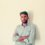# Simplify

0.6666...+0.77777...+0.88888....+.33333... yields the result:
a-2(3/10)
b-2(2/3)
c-2.353535...
d-5(3/10)Note by Mehdi Balti
5 years, 3 months ago

This discussion board is a place to discuss our Daily Challenges and the math and science related to those challenges. Explanations are more than just a solution — they should explain the steps and thinking strategies that you used to obtain the solution. Comments should further the discussion of math and science.

When posting on Brilliant:

• Use the emojis to react to an explanation, whether you're congratulating a job well done , or just really confused .
• Ask specific questions about the challenge or the steps in somebody's explanation. Well-posed questions can add a lot to the discussion, but posting "I don't understand!" doesn't help anyone.
• Try to contribute something new to the discussion, whether it is an extension, generalization or other idea related to the challenge.

MarkdownAppears as
*italics* or _italics_ italics
**bold** or __bold__ bold
- bulleted- list
• bulleted
• list
1. numbered2. list
1. numbered
2. list
Note: you must add a full line of space before and after lists for them to show up correctly
paragraph 1paragraph 2

paragraph 1

paragraph 2

[example link](https://brilliant.org)example link
> This is a quote
This is a quote
    # I indented these lines
# 4 spaces, and now they show
# up as a code block.

print "hello world"
# I indented these lines
# 4 spaces, and now they show
# up as a code block.

print "hello world"
MathAppears as
Remember to wrap math in $$ ... $$ or $ ... $ to ensure proper formatting.
2 \times 3 $2 \times 3$
2^{34} $2^{34}$
a_{i-1} $a_{i-1}$
\frac{2}{3} $\frac{2}{3}$
\sqrt{2} $\sqrt{2}$
\sum_{i=1}^3 $\sum_{i=1}^3$
\sin \theta $\sin \theta$
\boxed{123} $\boxed{123}$

Sort by:

$0.\dot{6} + 0.\dot{7} + 0.\dot{8} + 0.\dot{3} = \dfrac{6}{9} + \dfrac{7}{9} + \dfrac{8}{9} + \dfrac{3}{9} = \dfrac{6+7+8+3}{9} = \dfrac{24}{9} = \dfrac{8}{3} = 2\frac{2}{3}$

- 5 years, 3 months ago

Thanks buddy :)

- 5 years, 3 months ago

0.666...+0.777...+0.888...+0.3333...

let x=0.888..

100x=88.888..

minus the first two values

99x=88

x=(88/ 99 } =8/8

repeat steps for all values and you get

(2/3)+(7/9)+(8/9)+(1/3)

=(8/3)

=2(2/3)

Sorry don't know how to use LaTeX!

- 5 years, 3 months ago

Thank you :)

- 5 years, 3 months ago

b

- 5 years, 3 months ago

Brilliant solution, couldn't have thought of a better way to solve it.

- 5 years, 3 months ago

Yeap got it well :)Thanks all of you

- 5 years, 3 months ago

What you did there, I see it.

- 5 years, 3 months ago

Thanks dear :*

- 5 years, 3 months ago